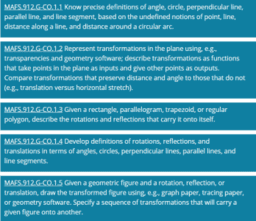# Congruence (CO) Cluster

MAFS.912.G-CO.1.1 Know precise definitions of angle, circle, perpendicular line, parallel line, and line segment, based on the undefined notions of point, line, distance along a line, and distance around a circular arc. MAFS.912.G-CO.1.2 Represent transformations in the plane using, e.g., transparencies and geometry software; describe transformations as functions that take points in the plane as inputs and give other points as outputs. Compare transformations that preserve distance and angle to those that do not (e.g., translation versus horizontal stretch). MAFS.912.G-CO.1.3 Given a rectangle, parallelogram, trapezoid, or regular polygon, describe the rotations and reflections that carry it onto itself. MAFS.912.G-CO.1.4 Develop definitions of rotations, reflections, and translations in terms of angles, circles, perpendicular lines, parallel lines, and line segments. MAFS.912.G-CO.1.5 Given a geometric figure and a rotation, reflection, or translation, draw the transformed figure using, e.g., graph paper, tracing paper, or geometry software. Specify a sequence of transformations that will carry a given figure onto another. MAFS.912.G-CO.2.6 Use geometric descriptions of rigid motions to transform figures and to predict the effect of a given rigid motion on a given figure; given two figures, use the definition of congruence in terms of rigid motions to decide if they are congruent. MAFS.912.G-CO.2.7 Use the definition of congruence in terms of rigid motions to show that two triangles are congruent if and only if corresponding pairs of sides and corresponding pairs of angles are congruent. MAFS.912.G-CO.2.8 Explain how the criteria for triangle congruence (ASA, SAS, SSS, and Hypotenuse-Leg) follow from the definition of congruence in terms of rigid motions. MAFS.912.G-CO.3.9 Prove theorems about lines and angles; use theorems about lines and angles to solve problems. Theorems include: vertical angles are congruent; when a transversal crosses parallel lines, alternate interior angles are congruent and corresponding angles are congruent; points on a perpendicular bisector of a line segment are exactly those equidistant from the segments endpoints. MAFS.912.G-CO.3.10 Prove theorems about triangles; use theorems about triangles to solve problems. Theorems include: measures of interior angles of a triangle sum to 180 triangle inequality theorem; base angles of isosceles triangles are congruent; the segment joining midpoints of two sides of a triangle is parallel to the third side and half the length; the medians of a triangle meet at a point. MAFS.912.G-CO.3.11 Prove theorems about parallelograms; use theorems about parallelograms to solve problems. Theorems include: opposite sides are congruent, opposite angles are congruent, the diagonals of a parallelogram bisect each other, and conversely, rectangles are parallelograms with congruent diagonals. MAFS.912.G-CO.4.12 Make formal geometric constructions with a variety of tools and methods (compass and straightedge, string, reflective devices, paper folding, dynamic geometric software, etc.). Copying a segment; copying an angle; bisecting a segment; bisecting an angle; constructing perpendicular lines, including the perpendicular bisector of a line segment; and constructing a line parallel to a given line through a point not on the line. MAFS.912.G-CO.4.13 Construct an equilateral triangle, a square, and a regular hexagon inscribed in a circle.